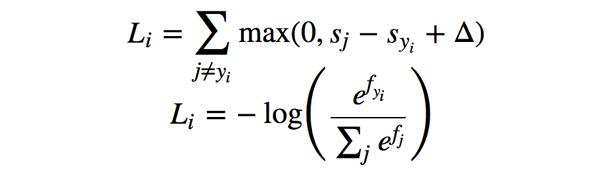## 原文如下

• 线性分类器简介
• 线性评分函数
• 阐明线性分类器
• 损失函数
• 多类SVM 译者注：中篇翻译截止处
• Softmax分类器
• SVM和Softmax的比较
• 基于Web的可交互线性分类器原型
• 小结

## 多类支持向量机损失 Multiclass Support Vector Machine Loss—————————————————————————————————————————

—————————————————————————————————————————def L_i(x, y, W):
"""
unvectorized version. Compute the multiclass svm loss for a single example (x,y)
- x is a column vector representing an image (e.g. 3073 x 1 in CIFAR-10)
with an appended bias dimension in the 3073-rd position (i.e. bias trick)
- y is an integer giving index of correct class (e.g. between 0 and 9 in CIFAR-10)
- W is the weight matrix (e.g. 10 x 3073 in CIFAR-10)
"""
delta = 1.0 # see notes about delta later in this section
scores = W.dot(x) # scores becomes of size 10 x 1, the scores for each class
correct_class_score = scores[y]
D = W.shape # number of classes, e.g. 10
loss_i = 0.0
for j in xrange(D): # iterate over all wrong classes
if j == y:
# skip for the true class to only loop over incorrect classes
continue
# accumulate loss for the i-th example
loss_i += max(0, scores[j] - correct_class_score + delta)
return loss_i

def L_i_vectorized(x, y, W):
"""
A faster half-vectorized implementation. half-vectorized
refers to the fact that for a single example the implementation contains
no for loops, but there is still one loop over the examples (outside this function)
"""
delta = 1.0
scores = W.dot(x)
# compute the margins for all classes in one vector operation
margins = np.maximum(0, scores - scores[y] + delta)
# on y-th position scores[y] - scores[y] canceled and gave delta. We want
# to ignore the y-th position and only consider margin on max wrong class
margins[y] = 0
loss_i = np.sum(margins)
return loss_i

def L(X, y, W):
"""
fully-vectorized implementation :
- X holds all the training examples as columns (e.g. 3073 x 50,000 in CIFAR-10)
- y is array of integers specifying correct class (e.g. 50,000-D array)
- W are weights (e.g. 10 x 3073)
"""
# evaluate loss over all examples in X without using any for loops
# left as exercise to reader in the assignment


## 实际考虑## 译者反馈

1. 允许转载，须全文转载并注明原文链接
2. 近期发现某些微信公众号转载时有删除贡献者们名字，或不注明原链接，或截取段落等不良转载行为，请停止以上不良转载行为，全文转载并注明原文链接。否则我们保留维权的权利，下一步将委托维权骑士进行版权保护；
3. 请知友们通过评论和私信等方式批评指正，贡献者均会补充提及。